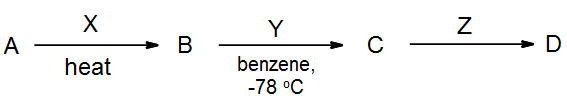# 7.1: Representing Organic Reactions

$$\newcommand{\vecs}{\overset { \rightharpoonup} {\mathbf{#1}} }$$ $$\newcommand{\vecd}{\overset{-\!-\!\rightharpoonup}{\vphantom{a}\smash {#1}}}$$$$\newcommand{\id}{\mathrm{id}}$$ $$\newcommand{\Span}{\mathrm{span}}$$ $$\newcommand{\kernel}{\mathrm{null}\,}$$ $$\newcommand{\range}{\mathrm{range}\,}$$ $$\newcommand{\RealPart}{\mathrm{Re}}$$ $$\newcommand{\ImaginaryPart}{\mathrm{Im}}$$ $$\newcommand{\Argument}{\mathrm{Arg}}$$ $$\newcommand{\norm}{\| #1 \|}$$ $$\newcommand{\inner}{\langle #1, #2 \rangle}$$ $$\newcommand{\Span}{\mathrm{span}}$$ $$\newcommand{\id}{\mathrm{id}}$$ $$\newcommand{\Span}{\mathrm{span}}$$ $$\newcommand{\kernel}{\mathrm{null}\,}$$ $$\newcommand{\range}{\mathrm{range}\,}$$ $$\newcommand{\RealPart}{\mathrm{Re}}$$ $$\newcommand{\ImaginaryPart}{\mathrm{Im}}$$ $$\newcommand{\Argument}{\mathrm{Arg}}$$ $$\newcommand{\norm}{\| #1 \|}$$ $$\newcommand{\inner}{\langle #1, #2 \rangle}$$ $$\newcommand{\Span}{\mathrm{span}}$$

Organic reactions are often shown in the following way:Reactions are not usually balanced; the above should be considered a scheme rather than an equation.  In fact, by-products are often omitted completely, especially if they are inorganic. A “reagent” is a chemical used to change an organic reactant into the desired product.  There may be several steps, listing more than one reaction in one scheme, by showing each reagent/condition in sequence after (i), (ii), etc.Here the alcohol is first treated with PBr3 and heated, then (in a separate reaction) it is treated with KCN in ethanol.

The value of this approach is seen when drawing out a multi-step synthesis.  In such a scheme, it shows a starting material A being acted upon by a sequence of reagents (X, Y, then Z) in order to turn it eventually into D:Notice that each arrow here represents a complete reaction, not just a step in a mechanism.